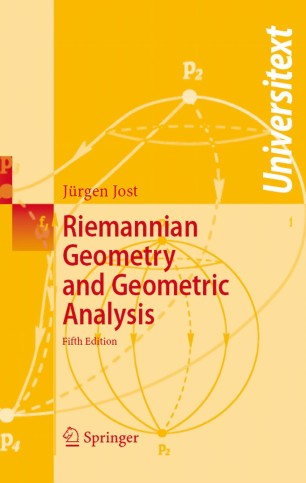# Riemannian Geometry and Geometric Analysis

• Jürgen JostTextbook

Part of the Universitext book series (UTX)

1. Front Matter
Pages i-xiii
2. Pages 1-85
3. Pages 179-241
4. Pages 243-299
5. Pages 301-391
6. Pages 393-468
7. Pages 469-519
8. Pages 521-543
9. Back Matter
Pages 545-583

### Introduction

This established reference work continues to lead its readers to some of the hottest topics of contemporary mathematical research.This new edition introduces and explains the ideas of the parabolic methods that have recently found such a spectacular success in the work of Perelman at the examples of closed geodesics and harmonic forms. It also discusses further examples of geometric variational problems from quantum field theory, another source of profound new ideas and methods in geometry.

From the reviews:

"This book provides a very readable introduction to Riemannian geometry and geometric analysis. The author focuses on using analytic methods in the study of some fundamental theorems in Riemannian geometry, e.g., the Hodge theorem, the Rauch comparison theorem, the Lyusternik and Fet theorem and the existence of harmonic mappings. With the vast development of the mathematical subject of geometric analysis, the present textbook is most welcome. [..] The book is made more interesting by the perspectives in various sections." Mathematical Reviews

### Keywords

Floer homology Kähler geometry Morse theory Riemannian geometry Seiber-Witten functionals curvature harmonic maps manifold symmetric spaces

#### Authors and affiliations

• Jürgen Jost
• 1
1. 1.Max Planck Institute for Mathematics in the Sciences04103Germany